##### Search### KCSE CLUSTER TESTS 23

#### Chemistry Paper 2

1.

a) The grid below represents a periodic table. Study it and answer the questions that follow. The letters do not represent the actual symbols of the elements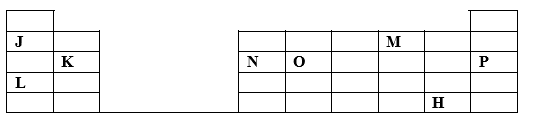i) Write the formula of the compound formed by element J and M.

ii) How does reactivity of K and N compare. Explain

iii) How does the atomic size of K and O compare. Explain.
b) What type of bonding exist in the chloride of L.

c) The following graph shows the reactivity of elements in period 3.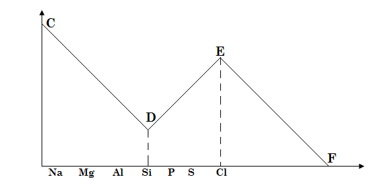Explain:
i) Trend CD

ii) Trend DE

d) The table below gives information on the m.pt of compounds of period 3 elements.
The letters do not represent the actual symbols.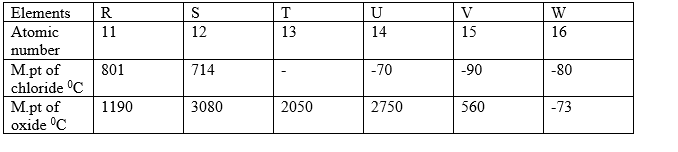I) a) Write the formula of: i) chloride of T

ii) Oxide of U V

II) Using the information above, suggest the type of bonding present in the chloride of

III) Explain:

i) The difference in m.pts of chloride and oxide of U in terms of structure.

ii) Why there is no m.pt in the chloride of T.

14 marks

2.

Study the flow chart below and answer the questions that follow.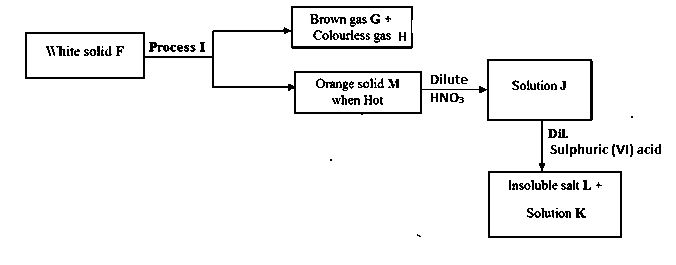a) Name substances

F

G

H

M

J

b) Name process I.

c) Write a balanced equation for the formation of G, H, and M.

d) Describe the test for the Brown gas G.

e) Write a balanced equation for the formation of J.

f) What is: Solution K Insoluble salt L. (½ mark)

g) Starting with copper metal, describe how a solid sample of copper II carbonate can be prepared.

13 marks

3.

The set up below was used to investigate the effect of electric current on lead II bromide.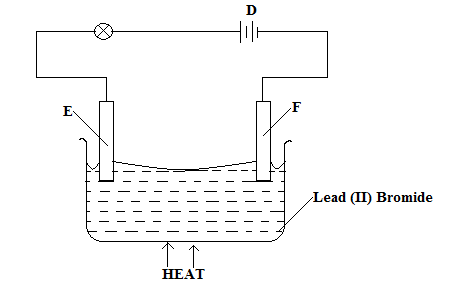a) Name the parts labelled

E

F

c) Write the equation for the reaction taking place at:

d) If heat is removed, the bulb goes off after sometime. Explain

e) Name the process being illustrated above.

f) Electroplating is one of the application of the process illustrated above. State any two reasons for electroplating.

9 marks

4.

Study the flow chart below and answer the questions that follow: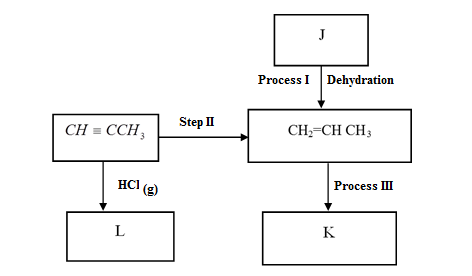i) Name substances J and L.

ii) State two conditions necessary for process I to take place.

iii) Name the reagent and conditions necessary for step II to occur.

Conditions

iv) Molecules of CH2 = CH2 polymerize to form a large molecule M whose structural formula is as follows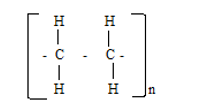State two uses of M.

v) i) What is an isomer?

ii) Draw and name two isomer of butene.

12 marks

5.

The diagram below is a representation for preparation of dilute nitric V acid on small scale. Study it and answer the questions that follow.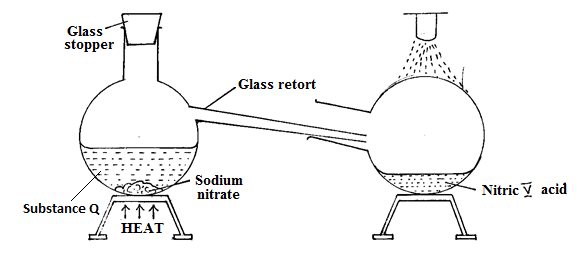a) (i) Give the name of substance Q.

(ii) Which other substance can be used in place of sodium nitrate.

b) Explain the following:

i) It is not advisable to use a stopper made of rubber in the above experiment.

ii) When dilute nitric (V) acid reacts with copper metal in open air it emits Brown fumes.

c) i) Name two sources of hydrogen gas in the haber process.

ii) A factory uses nitric (V) acid and ammonia in the preparation of a fertilizer. If the daily production of the fertilizer is 3200kg:

Calculate the mass of ammonia gas used in kg. (N =14.0; O =16.0, H=1.0).
iii) State two other uses of nitric (V) acid other than the production of fertilizer.

12 marks

6.

a) The table below gives the results of an experiment performed by Form 3 students from Kilimani High school.

Use it to answer the questions that follow.

Mass of crucible + Lid 19.52g

Mass of crucible + Lid + Magnesium ribbon 20.36g

Mass of crucible + Lid + Magnesium oxide 20.92g.

i) What is the percentage by mass of magnesium and oxygen in magnesium oxide as from the results.
ii) Determine the empirical formula of magnesium oxide.

b) i) 200cm3 of oxygen gas diffuses through a porous plate in 60 seconds. How long will it take 300cm3 of Sulphur IV oxide gas to diffuse through the same plate: (3marks)
ii) Which gas diffused faster? Explain

9 marks

7.

a) Below is a set up in the preparation of a particular salt. Study it and answer the questions that follow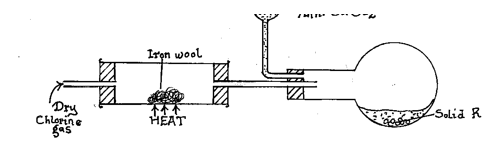i) What observation will be made in the combustion tube when dry hydrogen chloride gas is passed instead of dry chlorine. Explain

ii) Identify solid R.

iii) Give the property that makes solid R to be collected in the flask as shown above.

iv) State the purpose of anhydrous CaCl2 in the set up.

v) Give the name of two reagents that can be heated to produce chlorine gas.

vi) What would happen to solid R if dump chlorine gas was used in the above experiment.

vii) Calcium oxide would be preferred to anhydrous Calcium chloride in the above set-up. Explain

b) i) State and explain the observations that would be made when chlorine gas is mixed with hydrogen sulphide gas.

ii) Write an equation to show the bleaching action of chlorine water.

11 marks

Back Top